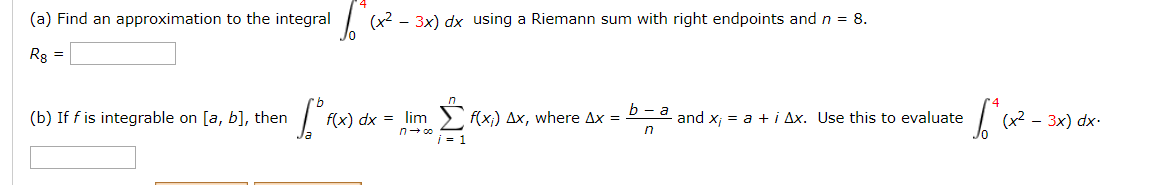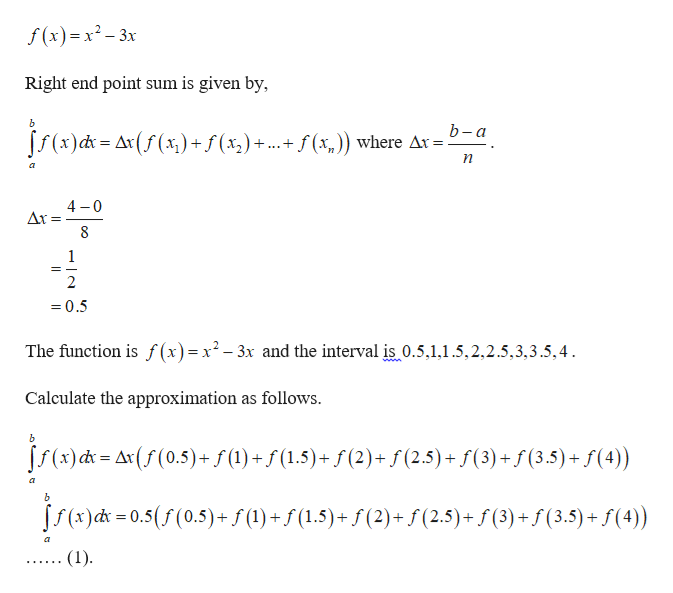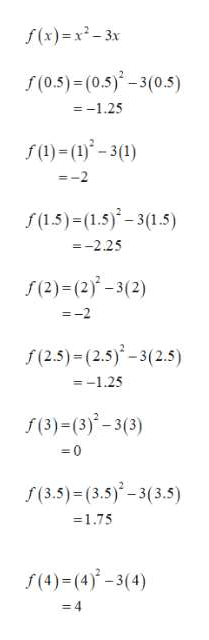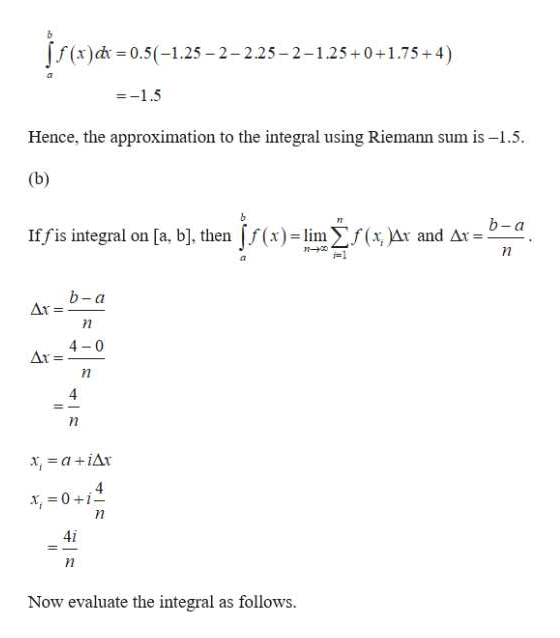# (a) Find an approximation to the integral(x2 - 3x) dx using a Riemann sum with right endpoints and n = 8.Rg =l.Σωκlim > f(x;) Ax, where Ax = P-a and x; = a +i Ax. Use this to evaluate(b) If f is integrable on [a, b], thenf(x) dx =3x) dx-

Question
10 viewshelp_outlineImage Transcriptionclose(a) Find an approximation to the integral (x2 - 3x) dx using a Riemann sum with right endpoints and n = 8. Rg = l.Σωκ lim > f(x;) Ax, where Ax = P-a and x; = a +i Ax. Use this to evaluate (b) If f is integrable on [a, b], then f(x) dx = 3x) dx- fullscreen
check_circle

Step 1

The given function is,help_outlineImage Transcriptionclosef() -x?— 3х Right end point sum is given by, [S(x)d = Ar(f (x) +f (x,)+ ...+ f (x,)) where Ar = 2 4 -0 Ar = = 0.5 The function is f (x)= x² – 3x and the interval is 0.5.1,1.5,2,2.5,3,3.5,4. www. Calculate the approximation as follows. S(x) d = Ax(f (0.5)+f (1) + f (1.5)+ f (2)+ f (2.5) + f(3) + f (3.5) + f(4)) S(x)dx = 0.5(f (0.5)+ f (1) + f (1.5)+ ƒ (2) + f (2.5)+ f (3) + f (3.5) + f(4)) (1). fullscreen
Step 2help_outlineImage Transcriptionclosef(x)=x²- 3x S(0.5) = (0.5)* -3(0.5) = -1.25 f (1) = (1)° - 3(1) =-2 f (1.5) = (1.5)-3(1.5) =-2.25 (2)=(2)* – 3(2) =-2 f(2.5) = (2.5)-3(2.5) = -1.25 f(3)=(3)-3(3) = 0 f(3.5) = (3.5) -3(3.5) =1.75 f(4)=(4)-3(4) = 4 fullscreen
Step 3

Substitute the above value in the...help_outlineImage Transcriptionclose[f(x)d = 0.5(-1.25 – 2-2.25-2-1.25+0+1.75+4) =-1.5 Hence, the approximation to the integral using Riemann sum is –1.5. (b) b-a If fis integral on [a, b], then f(x)= lim Ef(x Ar and Ar = b-a Ar = 4-0 Ar = 4 x, =a +iAr 4 X, = 0 +i 4i Now evaluate the integral as follows. fullscreen

### Want to see the full answer?

See Solution

#### Want to see this answer and more?

Solutions are written by subject experts who are available 24/7. Questions are typically answered within 1 hour.*

See Solution
*Response times may vary by subject and question.
Tagged in
MathCalculus

### Other